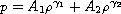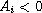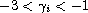Electron. J. Differential Equations, Vol. 2017 (2017), No. 156, pp. 1-18.

### Axisymmetric solutions of a two-dimensional nonlinear wave system with a two-constant equation of state Guodong Wang, Yanbo Hu, Huayong Liu

Abstract:
We study a special class of Riemann problem with axisymmetry for two-dimensional nonlinear wave equations with the equation of state,,(i=1,2). The main difficulty lies in that the equations can not be directly reduced to an autonomous system of ordinary differential equations. To solve it, we use the axisymmetry and self-similarity assumptions to reduce the equations to a decoupled system which includes three components of solution. By solving the decoupled system, we obtain the structures of the corresponding solutions and their existence.

Submitted February 17, 2017. Published June 28, 2017.
Math Subject Classifications: 35L65, 35J70, 35R35.
Key Words: Nonlinear wave system; generalized Chaplygin gas; axisymmetry; decoupled system.

Show me the PDF file (326 KB), TEX file for this article.Guodong Wang School of Mathematics and Physics Anhui Jianzhu University of China Hefei 230601, China email: gdwang@163.com Yanbo Hu Department of Mathematics Hangzhou Normal University of China Hangzhou 310036, China email: yanbo.hu@hotmail.com Huayong Liu School of Mathematics and Physics Anhui Jianzhu University of China Hefei 230601, China email: liuhy@ahjzu.edu.cn